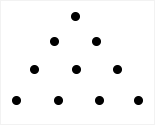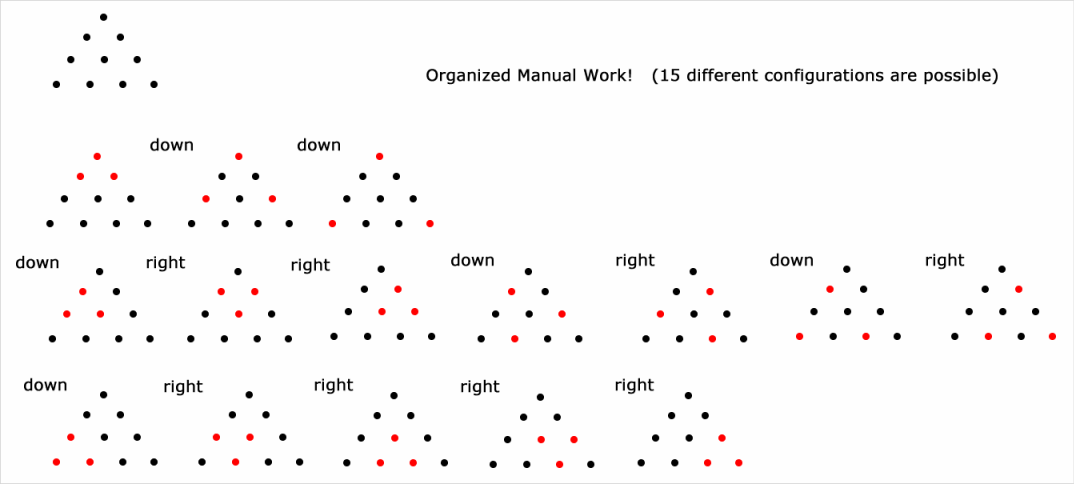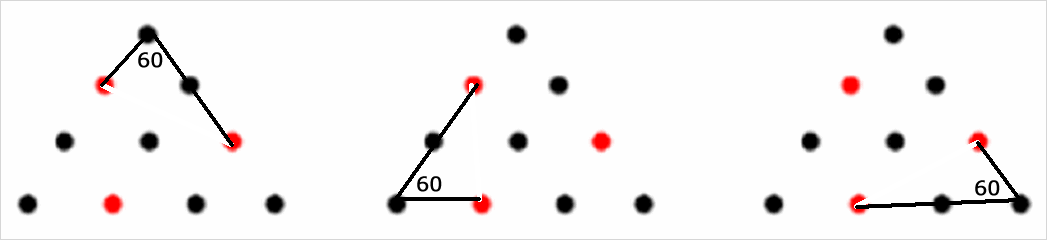GMAT Question of the Day - Daily to your Mailbox; hard ones only

 It is currently 15 Oct 2019, 00:28GMAT Club Daily Prep

Thank you for using the timer - this advanced tool can estimate your performance and suggest more practice questions. We have subscribed you to Daily Prep Questions via email.

Customized
for You

we will pick new questions that match your level based on your Timer History

Track

every week, we’ll send you an estimated GMAT score based on your performance

Practice
Pays

we will pick new questions that match your level based on your Timer History

Not interested in getting valuable practice questions and articles delivered to your email? No problem, unsubscribe here.From the figure shown, each of N people was asked to take 12 points ou

 new topic post reply Question banks Downloads My Bookmarks Reviews Important topics
Author Message
TAGS:

Hide Tags

GMATH TeacherP
Status: GMATH founder
Joined: 12 Oct 2010
Posts: 935
From the figure shown, each of N people was asked to take 12 points ou  [#permalink]

Show Tags00:00

Difficulty:95% (hard)

Question Stats:22% (02:45) correct78% (02:22) wrongbased on 27 sessions

HideShow timer Statistics

GMATH practice exercise (Quant Class 18)From the figure shown, each of N people will be asked to take 7 points out, so that the 3 points left form vertices of an equilateral triangle. If all of them proceed correctly, what is the minimum value of N to guarantee that at least two people will take out exactly the same 7 points?

(A) 13
(B) 14
(C) 15
(D) 16
(E) 17

_________________
Fabio Skilnik :: GMATH method creator (Math for the GMAT)
Our high-level "quant" preparation starts here: https://gmath.net
GMATH TeacherP
Status: GMATH founder
Joined: 12 Oct 2010
Posts: 935
Re: From the figure shown, each of N people was asked to take 12 points ou  [#permalink]

Show Tags

fskilnik wrote:
GMATH practice exercise (Quant Class 18)From the figure shown, each of N people will be asked to take 7 points out, so that the 3 points left form vertices of an equilateral triangle. If all of them proceed correctly, what is the minimum value of N to guarantee that at least two people will take out exactly the same 7 points?

(A) 13
(B) 14
(C) 15
(D) 16
(E) 17$$?\,\, = \,\,\left( {\# \,\,{\rm{possible}}\,\,\Delta \,\,{\rm{equilateral}}\,\,{\rm{configurations}}} \right)\,\,{\rm{ + }}\,\,{\rm{1}}$$

$$? = 15 + 1 = 16$$

The correct answer is (D).

We follow the notations and rationale taught in the GMATH method.

Regards,
Fabio.
_________________
Fabio Skilnik :: GMATH method creator (Math for the GMAT)
Our high-level "quant" preparation starts here: https://gmath.net
ManagerS
Joined: 28 Jan 2019
Posts: 97
Location: India
GMAT 1: 700 Q49 V36GPA: 4
WE: Manufacturing and Production (Manufacturing)
Re: From the figure shown, each of N people was asked to take 12 points ou  [#permalink]

Show Tags

fskilnik wrote:
fskilnik wrote:
GMATH practice exercise (Quant Class 18)From the figure shown, each of N people will be asked to take 7 points out, so that the 3 points left form vertices of an equilateral triangle. If all of them proceed correctly, what is the minimum value of N to guarantee that at least two people will take out exactly the same 7 points?

(A) 13
(B) 14
(C) 15
(D) 16
(E) 17$$?\,\, = \,\,\left( {\# \,\,{\rm{possible}}\,\,\Delta \,\,{\rm{equilateral}}\,\,{\rm{configurations}}} \right)\,\,{\rm{ + }}\,\,{\rm{1}}$$

$$? = 15 + 1 = 16$$

The correct answer is (D).

We follow the notations and rationale taught in the GMATH method.

Regards,
Fabio.

Hi, please explain why 1 is added?
_________________
"Luck is when preparation meets opportunity!"
GMATH TeacherP
Status: GMATH founder
Joined: 12 Oct 2010
Posts: 935
From the figure shown, each of N people was asked to take 12 points ou  [#permalink]

Show Tags

1
OhsostudiousMJ wrote:
Hi, please explain why 1 is added?

Sure, OhsostudiousMJ !

This is a trivial application of the Pigeonhole Principle.

There are 15 different possibilities, therefore if (at most) 15 people must choose one configuration each, you will still have the possibility of all 15 people choosing different configurations.

When 15+1 people are considered, at least one configuration must be chosen twice.

Regards,
Fabio.

P.S.: https://en.wikipedia.org/wiki/Pigeonhole_principle
_________________
Fabio Skilnik :: GMATH method creator (Math for the GMAT)
Our high-level "quant" preparation starts here: https://gmath.net
ManagerB
Joined: 23 Aug 2017
Posts: 117
Re: From the figure shown, each of N people was asked to take 12 points ou  [#permalink]

Show Tags

fskilnik Bunuel chetan2u
Hi could you please explain how are the 2 combinations (4th and 5th from left in the 2nd row of the seven diagrams) equilateral triangles?
GMATH TeacherP
Status: GMATH founder
Joined: 12 Oct 2010
Posts: 935
From the figure shown, each of N people was asked to take 12 points ou  [#permalink]

Show Tags

Debashis Roy wrote:
fskilnik Bunuel chetan2u
Hi could you please explain how are the 2 combinations (4th and 5th from left in the 2nd row of the seven diagrams) equilateral triangles?
Hi Debashis_Roy !

Thank you for your interest in my problem and also in my solution.In the figure above I present ONE of the diagrams you have asked about (for the other, the reasoning is the same).

Please note that I must explain to you why the purple segments (here unfortunately they appear as light grey) have the same lengths.

Reason: we have three congruent triangles (shown), by the SAS case (same two sides and intermediate angle) and the purple segments are (in all cases) opposite to the 60-degrees angle.

(We know it is a 60-degrees angle because of the one-one-one corresponding triangles... see the FIRST equilateral triangle presented in my previous solution, also the first and the last ones in the last row!)

If something is still unclear, please let me (us) know!

Regards and success in your studies,
Fabio.
_________________
Fabio Skilnik :: GMATH method creator (Math for the GMAT)
Our high-level "quant" preparation starts here: https://gmath.net
ManagerB
Joined: 23 Aug 2017
Posts: 117
Re: From the figure shown, each of N people was asked to take 12 points ou  [#permalink]

Show Tags

Thanks I got it!..Dint think of it though...GMATH TeacherP
Status: GMATH founder
Joined: 12 Oct 2010
Posts: 935
Re: From the figure shown, each of N people was asked to take 12 points ou  [#permalink]

Show Tags

Debashis Roy wrote:
Thanks I got it!..Dint think of it though...This explanation is (as you have realized) very GMAT-related, therefore it is important to be understood throughly... I am glad you did!_________________
Fabio Skilnik :: GMATH method creator (Math for the GMAT)
Our high-level "quant" preparation starts here: https://gmath.net
Math ExpertV
Joined: 02 Aug 2009
Posts: 7958
Re: From the figure shown, each of N people was asked to take 12 points ou  [#permalink]

Show Tags

Debashis Roy wrote:
fskilnik Bunuel chetan2u
Hi could you please explain how are the 2 combinations (4th and 5th from left in the 2nd row of the seven diagrams) equilateral triangles?

Hi,
it might seem to you but since all triangles or dots make equilateral triangles, even 4th and 5th are equilateral triangle.
Calculate it you will get it
..draw a triangle in 4th case by joining the points and check the attachment
AttachmentsIMG_20190304_195729.jpg [ 2.94 MiB | Viewed 334 times ]

_________________Re: From the figure shown, each of N people was asked to take 12 points ou   [#permalink] 04 Mar 2019, 07:29
Display posts from previous: Sort by

From the figure shown, each of N people was asked to take 12 points ou

 new topic post reply Question banks Downloads My Bookmarks Reviews Important topics

 Powered by phpBB © phpBB Group | Emoji artwork provided by EmojiOne